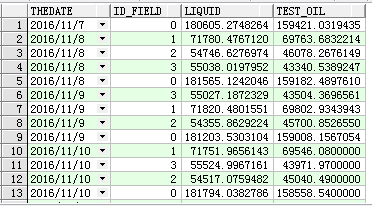select t.the__date,t.id__field,t.liquid,t.test__oil,__
--月累计
(select sum(t1.liquid) from TJ_FIELD_PROD_DAILY t1 where t1.id_field=t.id_field and t1.thedate<=t.thedate and t1.thedate>=(select trunc(t.thedate,'mm') from dual)),
(select sum(t1.test_oil) from TJ_FIELD_PROD_DAILY t1 where t1.id_field=t.id_field and t1.thedate<=t.thedate and t1.thedate>=(select trunc(t.thedate,'mm') from dual)),
--年累计
(select sum(t1.liquid) from TJ_FIELD_PROD_DAILY t1 where t1.id_field=t.id_field and t1.thedate<=t.thedate and t1.thedate>=(select trunc(t.thedate,'yyyy') from dual)),
(select sum(t1.test_oil) from TJ_FIELD_PROD_DAILY t1 where t1.id_field=t.id_field and t1.thedate<=t.thedate and t1.thedate>=(select trunc(t.thedate,'yyyy') from dual))
from TJ_FIELD_PROD_DAILY t

2个回答

select t.the__date,t.id__field,t.liquid,t.test__oil,__
--月累计
(select sum(t1.liquid),sum(t1.test_oil) from TJ_FIELD_PROD_DAILY t1 where t1.id_field=t.id_field and t1.thedate<=t.thedate and t1.thedate>=(select trunc(t.thedate,'mm') from dual)),
--年累计
(select sum(t1.liquid),sum(t1.test_oil) from TJ_FIELD_PROD_DAILY t1 where t1.id_field=t.id_field and t1.thedate<=t.thedate and t1.thedate>=(select trunc(t.thedate,'yyyy') from dual))
from TJ_FIELD_PROD_DAILY t